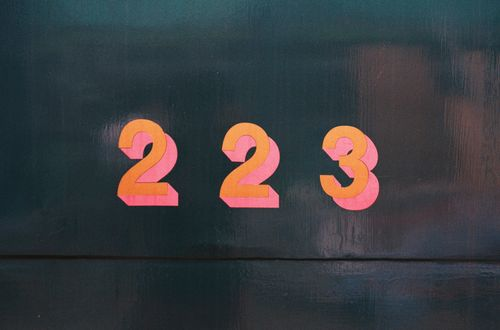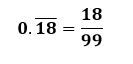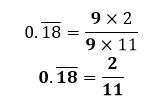# How to convert recurring decimals into fractions

Want to find out how to convert recurring decimals into fractions? We've provided a step-by-step guide of how to convert recurring decimals into fractions to teach you everything you need to know.## Step 1: Determine the number of recurring decimals

To convert 0.(87), we can count the number of repeating digits which are 8 and 9.

## Step 2: Express the recurring decimal as a fraction

Write the recurring decimal as a fraction by writing the whole number as the numerator. Write the denominator consisting of a series of 9s depending on the number of recurring digits.

## Step 3: Simplify the fractions

Simplify the fractions by cancelling out common factors shared by the numerator and denominator.

## Examples of how to convert recurring decimals into fractions

Q1) What is 0.(18) expressed in fraction?

For 0.(18), we can see that it has two recurring digits. To express it into a fraction, the denominator has two 9s.

We write 18 as the numerator and the denominator be 99. Hence, we have:Cancel out common factors and simplify the fraction.#### Join today

The fastest way to practice

Unlock our complete testing platform and improve faster that ever.